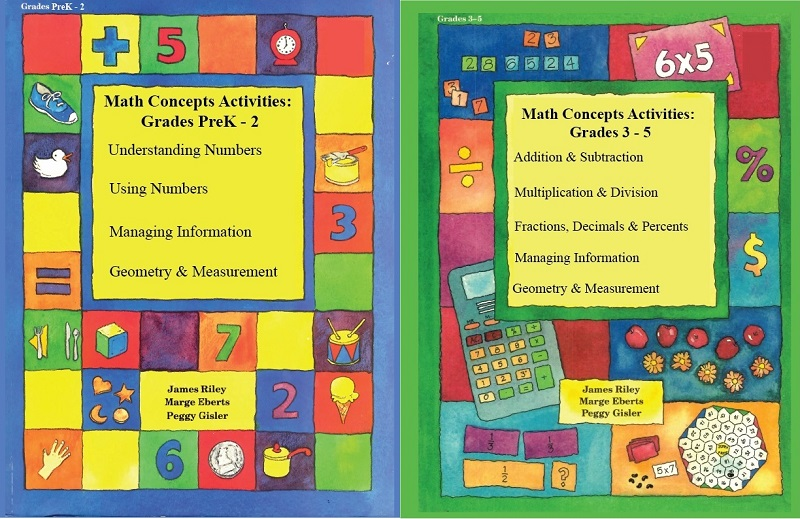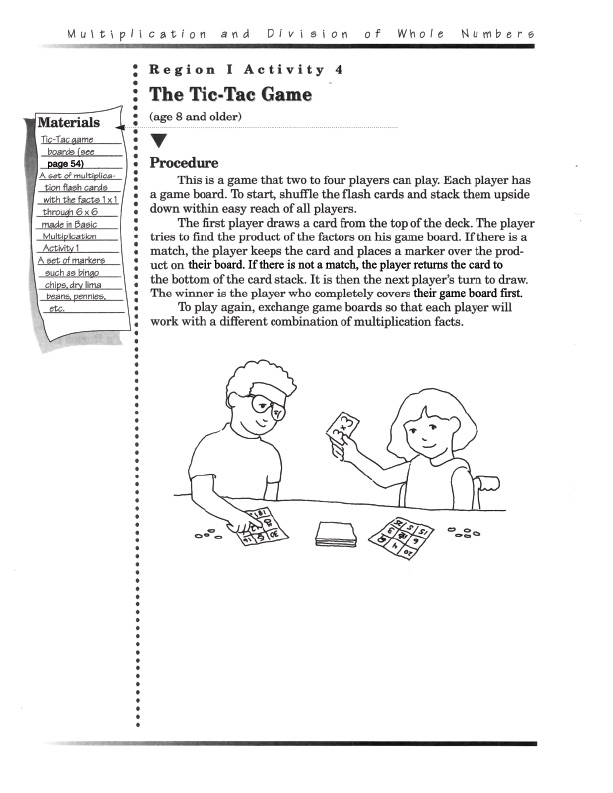Math Concepts ActivitiesYour children will have fun learning basic math concepts with activities using household items that will give them the solid math foundation that they need. Math teachers endorse the hands-on learning approach as the way to learn math concepts.

Using The Activities

• Parents can start on any topic
• Each activity has step-by-step instructions
• Math concepts are taught before procedures are introduced
• Activities are designed to be self-correcting so mistakes don't have to be pointed out

The Benefits of Hands-On Approach

• These activities challenge  children to use their imagination and creativity in developing an understanding of basic mathematical concepts and finding solutions to problems
• These activities involve parents in their children's efforts to learn math

Sample Activity